# Numerical methods for 1D ODEs

Consider the IVP: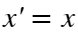, with the initial condition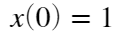.
We want to compute the numerical approximation for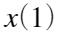, that is, to compute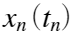for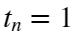using different numerical methods.

## Euler's method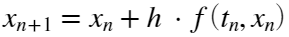where h is the time step.
Notice, from the numerical results below, that the error is proportional to.
We will approximate the value using N=10, 100, 1000, 10000 subdivisions
clear all;
f=@(t,x) x;
sol=exp(1); %we know the analytical solution
tini = 0;
xini = 1; %x(0)=1
tfin = 1;
N = [10,100,1000,10000];
t(1) = tini;
x(1) = xini;
for k = 1:4
h = (tfin-tini)/N(k);
npoints = N(k)+1;
for i=2:npoints
x(i) = x(i-1)+h*f(t(i-1),x(i-1));
t(i)= t(i-1)+h;
end
fprintf('for N= %d, value x(1)= %14.12f error=%20.12e\n',N(k),x(end),abs(x(end)-exp(1)));
end
for N= 10, value x(1)= 2.593742460100 error= 1.245393683590e-01 for N= 100, value x(1)= 2.704813829422 error= 1.346799903752e-02 for N= 1000, value x(1)= 2.716923932236 error= 1.357896223150e-03 for N= 10000, value x(1)= 2.718145926825 error= 1.359016338189e-04

## Mid point Method

Similar to the Euler method we can use the midpoint method:Notice, from the numerical results below, that the error is proportional to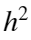.
clear x t;
t(1) = tini;
x(1) = xini;
for k = 1:4
h = (tfin-tini)/N(k);
npoints = N(k)+1;
for i=2:npoints
x(i) = x(i-1)+h*f(t(i-1)+0.5*h,x(i-1)+0.5*h*f(t(i-1),x(i-1)));
t(i) = t(i-1)+h;
end
fprintf('for N= %d, value x(1)= %14.12f error=%20.12e\n',N(k),x(end),abs(x(end)-exp(1)));
end
for N= 10, value x(1)= 2.714080846608 error= 4.200981850822e-03 for N= 100, value x(1)= 2.718236862560 error= 4.496589908864e-05 for N= 1000, value x(1)= 2.718281375752 error= 4.527072827720e-07 for N= 10000, value x(1)= 2.718281823929 error= 4.530157582394e-09

## Implicit Euler Method

The implicit or backward Euler's method consists in taken the slope at the next point (already unknown).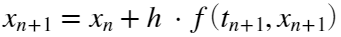This is an implicit equation on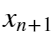. Because of that, unless any other information or simplification about the ODE is know, you have to solve a non-linear numerical equation at each time step.
An usual approach is to approximate using taylor expansionIf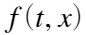does'nt depends on t,. Therefore only the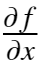is computed and thenand using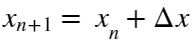, then we obtain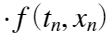that must be solved for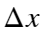.
For the exemple we are dealing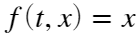and thereforeandThus, the implicit Euler method for this exemple can be written as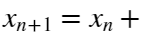.
Exercise: Build the Matlab code for this example and compute the results for N=10, 100, 1000, 10000 subdivisions.

## Runge-Kutta Methods

Runge-Kutta 2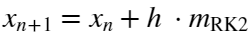withwhere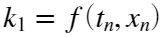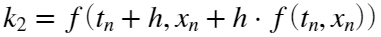Exercise: Build the Matlab code for this example and compute the results for N=10, 100, 1000, 10000 subdivisions.
Notice, from the numerical results below, that the error is proportional to.
Runge-Kutta 4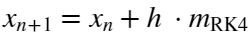withwhere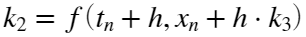Exercise: Build the Matlab code for this example and compute the results for N=10, 100, 1000, 10000 subdivisions.
Notice, from the numerical results below, that the error is proportional to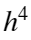.

## Home work:

Exercise1: Build the Euler Method as a Matlab function defined by
function [ t, X ] = eulerMethod ( f, timeRange, xini, N)
input:
f: function address for the differential equations
timeRange=[ tini, tfin ]: time interval 1x2 vector
xini: initial values nx1 vector (n the dimension of the problem)
N: number of subdivisions
output:
t: vector with all the time steps. t(1) = tini; t(end) =tfin;
X: trajectory with all the computed points, (N+1)xn Matrix
Exercise 2:
Do the same with the Mid Point Method as a Matlab function defined by
function [ t, X ] = midPointMethod ( f, timeRange, xini, N)
Exercise 3:
Do the same with the RK2 Method as a Matlab function defined by
function [ t, X ] = RK2Method ( f, timeRange, xini, N)
Exercise 4:
Do the same with the RK4 Method as a Matlab function defined by
function [ t, X ] = RK4Method ( f, timeRange, xini, N)
Exercise 5:
Apply all these methods to the solve the ODEfor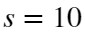and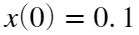and compare with the exact solutionwhere